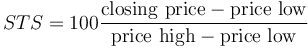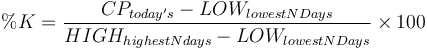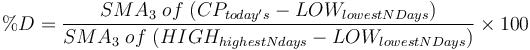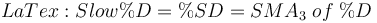The stochastic oscillator is a momentum indicator used in technical analysis of stocks, introduced by George Lane in the 1950s, to compare the closing price of a commodity to its price range over a given time span.

This indicator is usually calculated as:$STS = 100 \frac{\text{closing price} - \text{price low}}{\text{price high} - \text{price low}}$

and can be manipulated by changing the period considered for highs and lows.

The idea behind this indicator is that prices tend to close near their past highs in bull markets, and near their lows in bear markets. Transaction signals can be spotted when the stochastic oscillator crosses its moving average.

Two stochastic oscillator indicators are typically calculated to assess future variations in prices, a fast (%K) and slow (%D). Comparisons of these statistics are a good indicator of speed at which prices are changing or the Impulse of Price. %K is the same as Williams %R, though on a scale 0 to 100 instead of -100 to 0, but the terminology for the two are kept separate.

## Fast Stochastic vs Slow Stochastic

The fast stochastic oscillator (or Stoch %K) calculates the ratio of two closing price statistics: the difference between the latest closing price and the lowest price in the last N days over the difference between the highest and lowest prices in the last N days:$\%K = { CP_{today's} - LOW_{lowestNDays} \over HIGH_{highestNdays} - LOW_{lowestNDays} } \times 100$
Where:
CP is closing price
LOW is low price
HIGH is high price

The usual "N" is 14, 9 or 5 days but this can be varied. When the current closing price is the low for the last N-days, the %K value is 0, when the current closing price is a high for the last N-days, %K=100.

The slow stochastic oscillator (or Stoch %D) calculates the simple moving average of the Stoch %K statistic across s periods . Usually s=3:$\%D = { SMA_3 \; of \; ( CP_{today's} - LOW_{lowestNDays} ) \over SMA_3 \; of \; ( HIGH_{highestNdays} - LOW_{lowestNDays} ) } \times 100$$LaTex: Slow\%D = \%SD = SMA_3 \; of \; \%D$

The %K and %D oscillators range from 0 to 100 and are often visualized using a line plot. Levels near the extremes 100 and 0, for either %K or %D, indicate strength or weakness (respectively) because prices have made or are near new N-day highs or lows.

## Applications of Fast and Slow Stochastic

There are two well known methods for using the %K and %D indicators to make decisions about when to buy or sell stocks. The first involves crossing of %K and %D signals, the second involves basing buy and sell decisions on the assumption that %K and %D oscillate.

In the first case, %D acts as a trigger or signal line for %K. A buy signal is given when %K crosses up through %D, or a sell signal when it crosses down through %D. Such crossovers can occur too often, and to avoid repeated whipsaws one can wait for crossovers occurring together with an overbought/oversold pullback, or only after a peak or trough in the %D line. If price volatility is high, a simple moving average of the Stoch %D indicator may be taken. This statistic smooths out rapid fluctuations in price.

In the second case, some analysts argue that %K or %D levels above 80 and below 20 can be interpreted as overbought or oversold. On the theory that the prices oscillate, many analysts including George Lane, recommend that buying and selling be timed to the return from these thresholds. In other words, one should buy or sell after a bit of a reversal. Practically, this means that once the price exceeds one of these thresholds, the investor should wait for prices to return through those thresholds (e.g. if the oscillator were to go above 80, the investor waits until it falls below 80 to sell).

The third way that traders will use this indicator is to watch for divergences where the Stochastic trends in the opposite direction of price. As with the RSI this is an indication that the momentum in the market is waning and a reversal may be in the making. For further confirmation many traders will wait for the cross below the 80 or above the 20 line before entering a trade on divergence. The chart below illustrates an example of where a divergence in stochastics relative to price forecasted a reversal in the price's direction.